# NEET Physics Kinematics (Graphical Questions)

Home » NEET Physics » NEET Physics Kinematics (Graphical Questions)

Here we are providing NEET Physics Graphical Questions for Kinematics. Most of the time students gets confused while solving graphical questions based on kinematics. we have provided enough problems here so that students can master the concepts of graph. This will help students to understand the concept well.

## NEET Physics Kinematics (Graphical Questions)

Question 1:Solution:Question 2:Solution:

(a) The slope of displacement-time graph goes on decreasing, it means the velocity is decreasing i.e. It’s motion is retarded and finally slope becomes zero i.e. particle stops.

Question 3:Solution:

(d) In the positive region the velocity decreases linearly (during rise) and in the negative region velocity increases linearly (during fall) and the direction is opposite to each other during rise and fall, hence fall is shown in the negative region.

Question 4: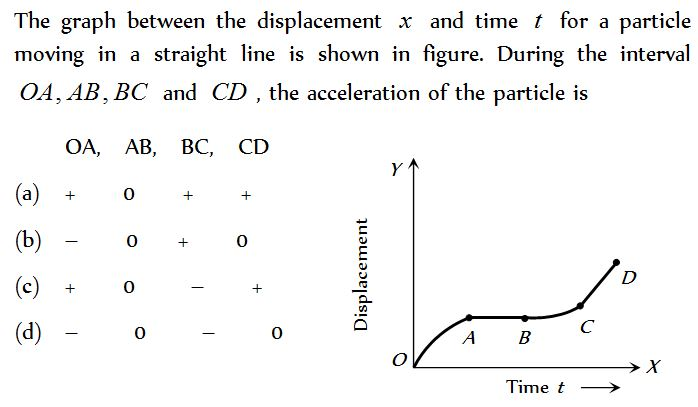Solution:

(b) Region OA shows that graph bending toward time axis e. acceleration is negative.

Region AB shows that graph is parallel to time axis i.e. velocity is zero. Hence acceleration is zero.

Region BC shows that graph is bending towards displacement axis i.e. acceleration is positive.

Region CD shows that graph having constant slope i.e. velocity is constant. Hence acceleration is zero.

Question 5: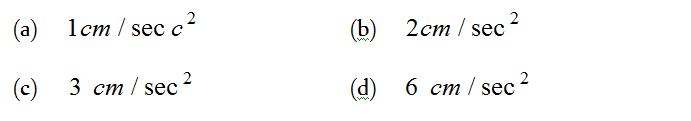Solution:Question 6:Solution:

(c) In part cd displacement-time graph shows constant slope e. velocity is constant. It means no acceleration or no force is acting on the body.

Question 7: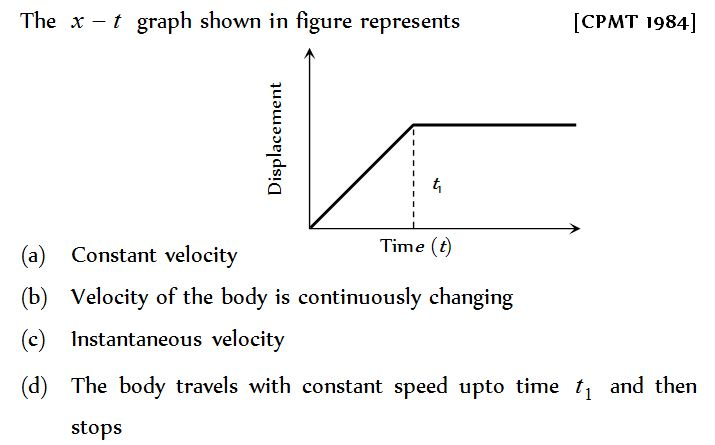Solution:Question 8:Solution: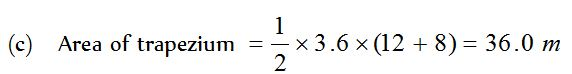Question 9:Solution:Question 10: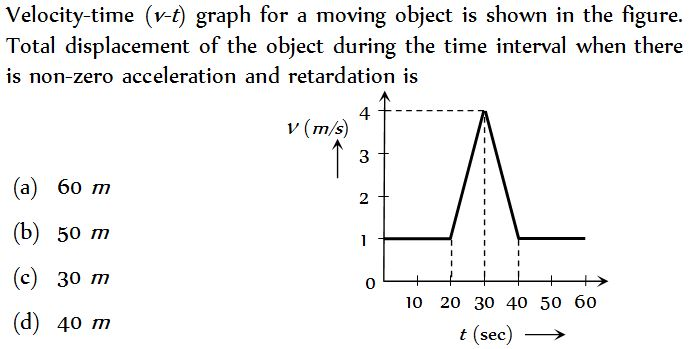Solution: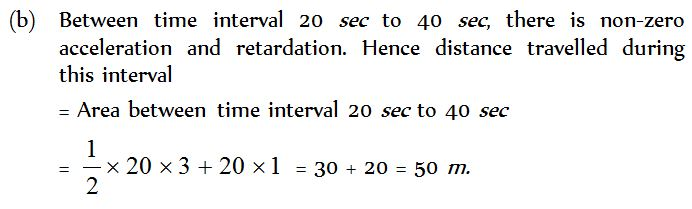Question 11:Solution: (c)

Question 12: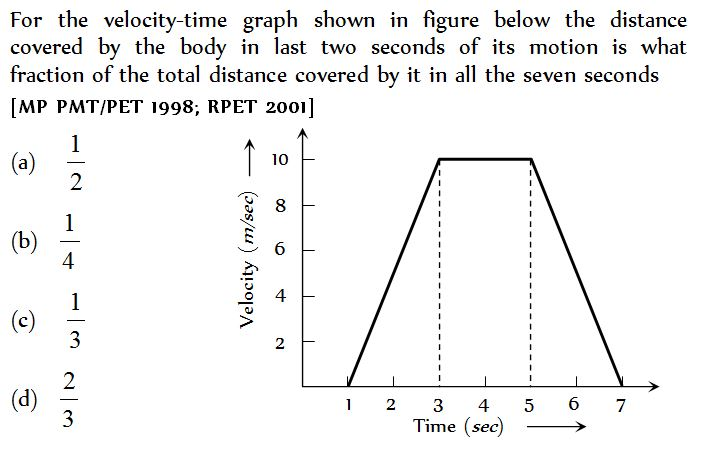Solution: (b)

Question 13:Solution:Question 14:Solution: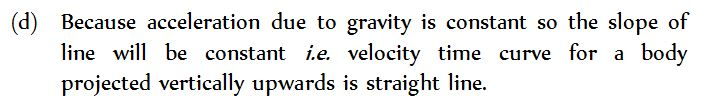Question 15: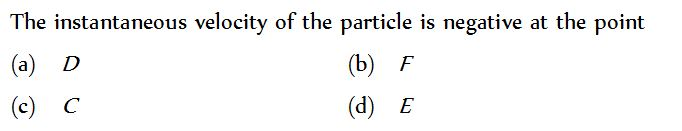Solution:Question 16: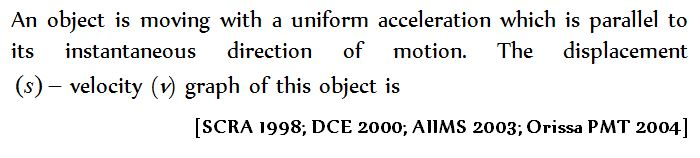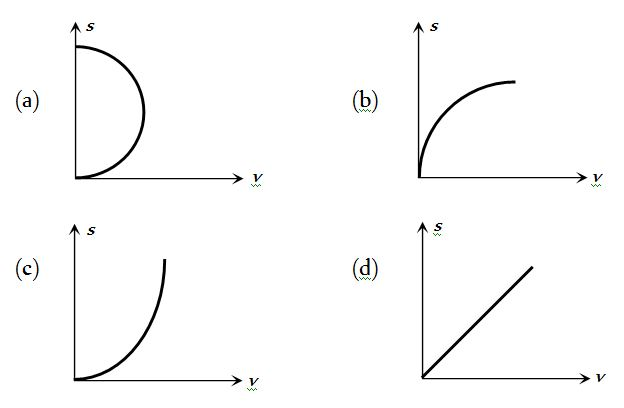Solution:Question 17: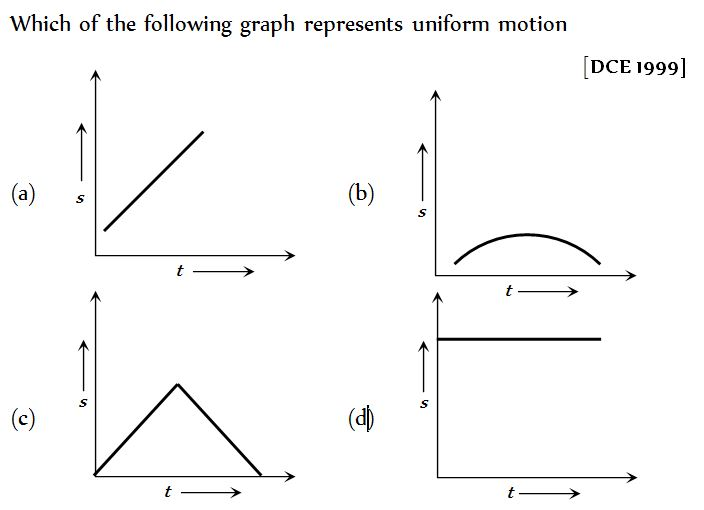Solution: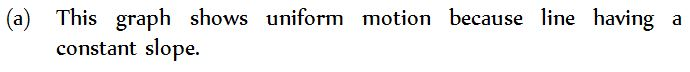Question 18:Solution:Question 19: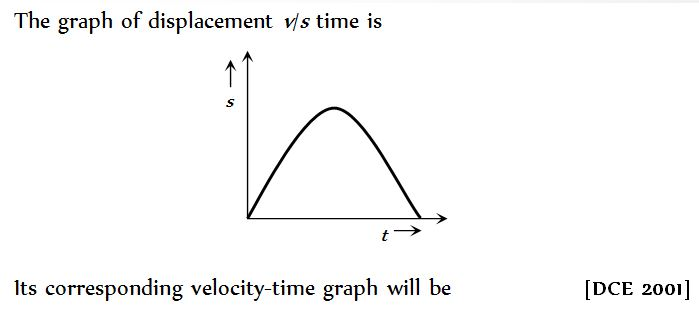Solution:Question 20:Solution: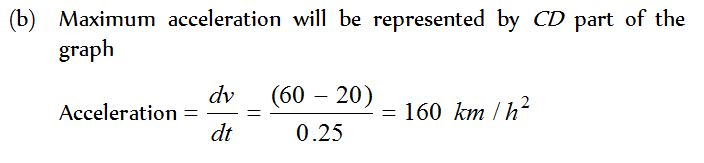Question 21:Solution: (d)

Question 22:Solution: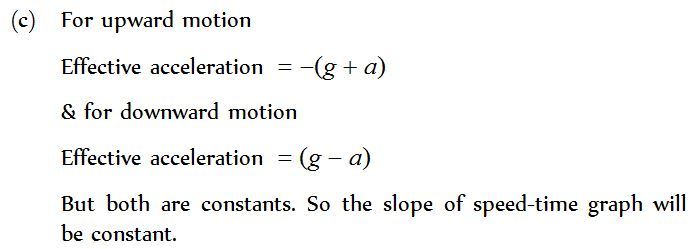Question 23: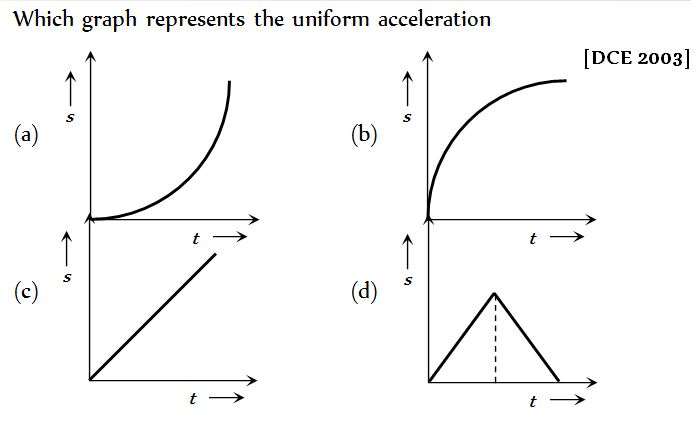Solution: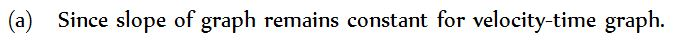Question 24:Solution: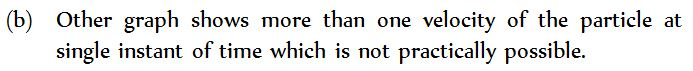Question 25: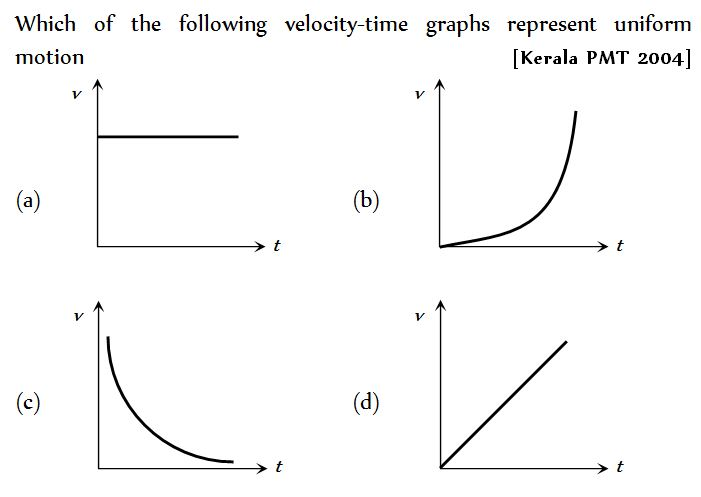Solution: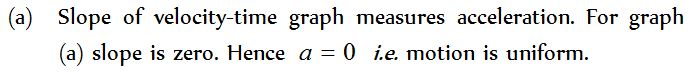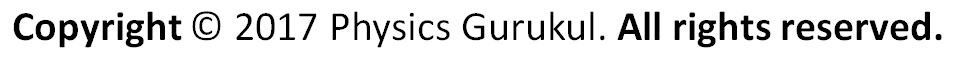One App For All Your Needs.

Announcements

Join our Online JEE Test Series for 499/- Only (Web + App) for 1 Year

Join our Online NEET Test Series for 499/- Only for 1 Year